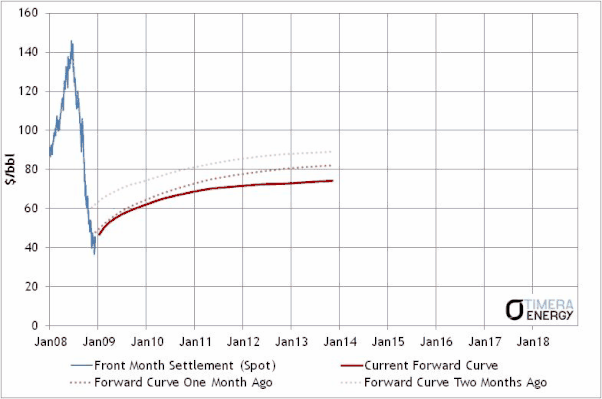Choose Language Hide Translation Bar
Highlighted

## Animated Forward Curve Graph

May I ask whether JMP allows me to build an animated forward curve graph as attached.

https://timera-energy.com/the-dangers-of-mixing-forecasts-and-forward-curves/3 REPLIES 3
Highlighted

## Re: Animated Forward Curve Graph

The 'animation' part is easy as this script shows. But here the 'forward curve' is just a straight line, but you would need to look into how that's actually calculated:

``````NamesDefaultToHere(1);

// Make some data
dt = NewTable("Data", NewColumn("Value", Numeric, Continuous, Formula(RandomNormal(0,1))));

// Graph the data (this script was built by JMP, apart from the 'add graphics script' part)
dt << Graph Builder(
Size( 535, 467 ),
Show Control Panel( 0 ),
Variables( X( Transform Column( "Row", Formula( Row() ) ) ), Y( :Value ) ),
Elements( Line( X, Y, Legend( 8 ) ) ),
SendToReport(
Dispatch(
{},
"Row",
ScaleBox,
{Min( 0 ), Max( 40 ), Inc( 2 ), Minor Ticks( 1 )}
),
Dispatch(
{},
"Value",
ScaleBox,
{Min( 3 ), Max( -3 ), Inc( 1 ), Minor Ticks( 0 )}
),
Dispatch(
{},
"Graph Builder",
FrameBox,
2,
Description( "Script" ),
Pen Color( "Red" );
Line Style( "Dotted" );
dt = Data Table( "Data" );
Line( {N Row( dt ), :Value[N Row( dt )]}, {40, 0} );
), Grid Line Order( 1 ), Reference Line Order( 3 )}
)
)
);

for(p=11, p<=40, p++, Wait(0.1); dt << addRow(1));``````
Highlighted

## Re: Animated Forward Curve Graph

Hi Ian

Thank you very much.

I will experiment with it and see where it leads...much appreciated...

Highlighted

## Re: Animated Forward Curve Graph

Hi Ian

Further to your helpful response, I am wondering whether the script need to modified to fit this price dataset.  Essentially the x-axis is the trading date and the Y-axis are  the spot month (represent the price graph) and M01 to M24 months (represent the forward curve "line" for 1st to 24th month prices as it work through the time series.)

I know my request may came across a bit imposing.  As I am a newbie on JMP graph builder; I was hoping that if your time permits, you could help me to set that into a JMP dataset and scripts, Through JMP, I could learn the way you did it and i will work through the iterations.  It is also okay if you are not able to act on my request if it is not appropriate.

Article Labels

There are no labels assigned to this post.